# Detection of space-time perturbations with quantum-enhanced metrology

Quiñones Valles, Diego A. (2017) Detection of space-time perturbations with quantum-enhanced metrology. PhD thesis, University of Leeds.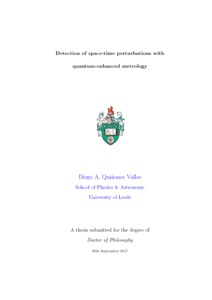Preview
Text (D. A. Quinones Phd Thesis)
PhD_thesis.pdf - Final eThesis - complete (pdf)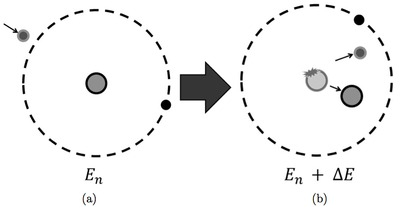Preview
Image (Figure 2.1)
atob.png - Final eThesis - complete (pdf)Image (Figure 2.2)
dnucleus.eps - Final eThesis - complete (pdf)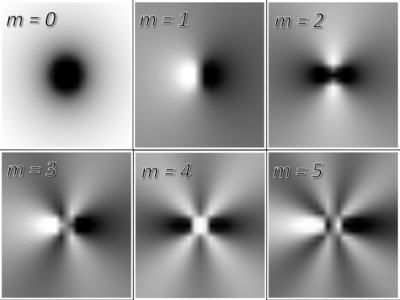Preview
Image (Figure 2.3)
spect.png - Final eThesis - complete (pdf)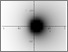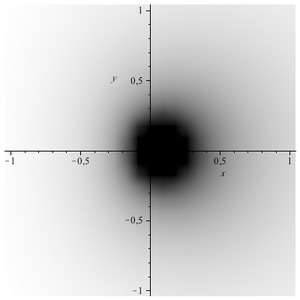Preview
Image (Figure 2.4)
spectsu.png - Final eThesis - complete (pdf)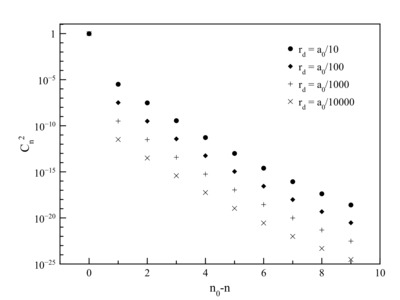Preview
Image (Figure 3.1)
cnvsn.png - Final eThesis - complete (pdf)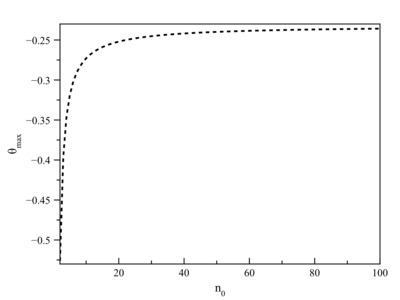Preview
Image (Figure 3.2)
thetavsn0.png - Final eThesis - complete (pdf)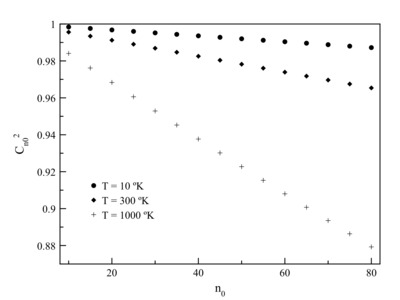Preview
Image (Figure 3.3)
cn0vsn0.png - Final eThesis - complete (pdf)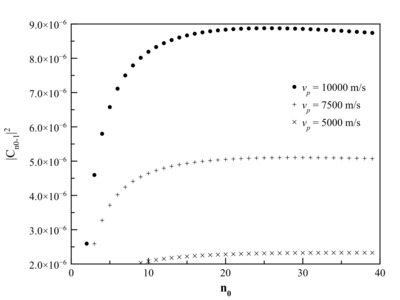Preview
Image (Figure 3.4)
cn01vsn0.png - Final eThesis - complete (pdf)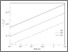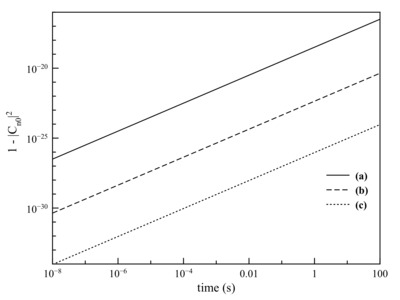Preview
Image (Figure 3.5)
cn0vst.png - Final eThesis - complete (pdf)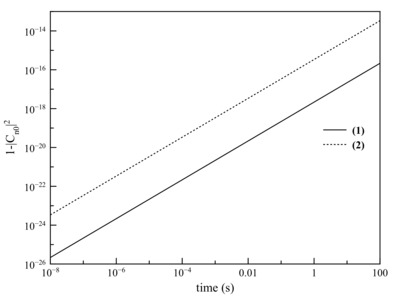Preview
Image (Figure 3.6)
cn0vst2.png - Final eThesis - complete (pdf)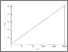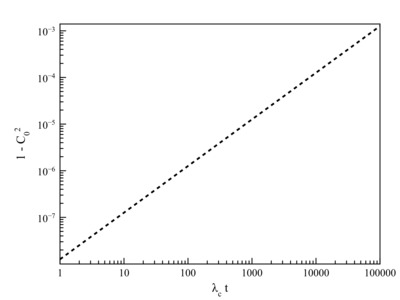Preview
Image (Figure 4.1)
cvlct.png - Final eThesis - complete (pdf)Image (Figure 4.2)
pic1.eps - Final eThesis - complete (pdf)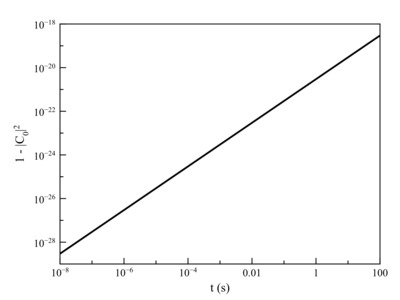Preview
Image (Figure 4.3)
c0vtscat.png - Final eThesis - complete (pdf)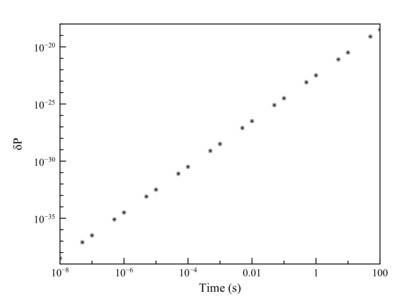Preview
Image (Figure 4.4)
rabiN.png - Final eThesis - complete (pdf)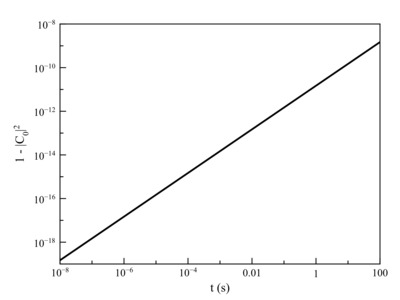Preview
Image (Figure 4.5)
c0vtgN.png - Final eThesis - complete (pdf)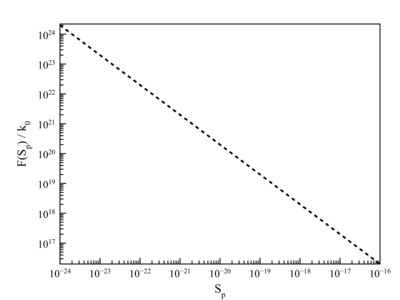Preview
Image (Figure 5.1)
fk000.png - Final eThesis - complete (pdf)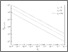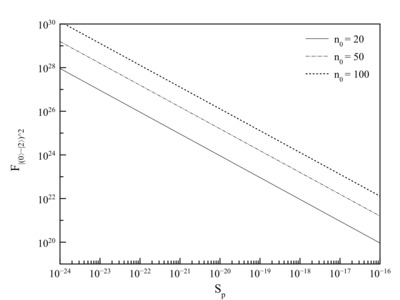Preview
Image (Figure 5.2)
f02_2.png - Final eThesis - complete (pdf)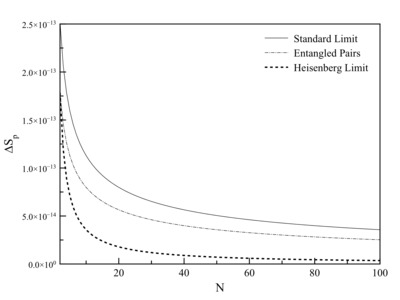Preview
Image (Figure 5.3)
dsp_n.png - Final eThesis - complete (pdf)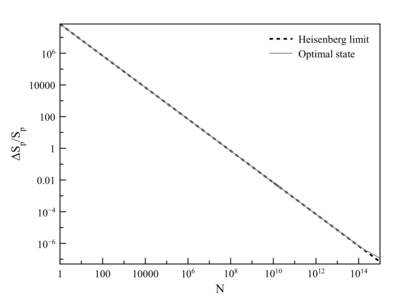Preview
Image (Figure 5.4)
dltsphp0.png - Final eThesis - complete (pdf)